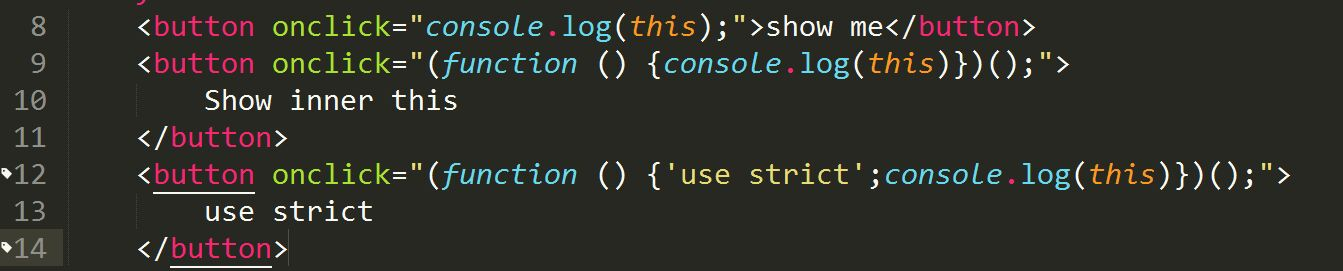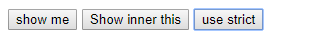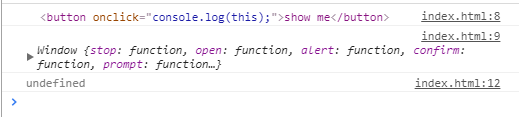# this指向

「从零单排canal 06」 instance模块源码解析

# 前言

## 全局环境下

``````console.log(this.document === document); // true

// 在浏览器中，全局对象为 window 对象：
console.log(this === window); // true

this.a = 37;
console.log(window.a); // 37
``````

## 函数上下文调用

### 函数直接调用

``````function f1(){
return this;
}

f1() === window; // true
``````

``````function f2(){
"use strict"; // 这里是严格模式
return this;
}

f2() === undefined; // true
``````

### 对象中的this

1.函数的定义无法确定this的指向，this指向只与调用函数的对象有关
2.多层嵌套的对象，内部方法的this指向离被调用函数最近的对象

``````var o = {
a:10,
b:{
a:12,
fn:function(){
console.log(this.a);//12
}
}
}
o.b.fn();
``````
``````var o = {
a:10,
b:{
a:12,
fn:function(){
console.log(this.a); //undefined
console.log(this); //window
}
}
}
var j = o.b.fn;
j();
``````

### 原型链中的this

``````var o = {
f : function(){
return this.a + this.b;
}
};
var p = Object.create(o);
p.a = 1;
p.b = 4;

console.log(p.f()); // 5
``````

## 构造函数中的this

``````function Fn(){
this.user = "jjj";
}
var a = new Fn();
console.log(a.user); //jjj
``````

### 构造函数中有return时

``````function Fn(){
this.user = "jjj";
return { user: 'aaa' }
}
var a = new Fn();
console.log(a.user); //aaa
``````
``````function fn()
{
this.user = 'jjj';
return {};
}
var a = new fn;
console.log(a.user); //undefined
``````
``````function fn()
{
this.user = 'jjj';
return function(){};
}
var a = new fn;
console.log(a.user); //undefined
``````
``````function fn()
{
this.user = 'jjj';
return 1;
}
var a = new fn;
console.log(a.user); //jjj
``````
``````function fn()
{
this.user = 'jjj';
return undefined;
}
var a = new fn;
console.log(a.user); //jjj
``````
``````function fn()
{
this.user = 'jjj';
return undefined;
}
var a = new fn;
console.log(a); //fn {user: "jjj"}
``````

``````function fn()
{
this.user = 'jjj';
return null;
}
var a = new fn;
console.log(a.user); //jjj
``````

## DOM事件处理函数

``````// 被调用时，将关联的元素变成蓝色
function bluify(e){
console.log(this); //在控制台打印出所点击元素
e.stopPropagation();  //阻止时间冒泡
e.preventDefault(); //阻止元素的默认事件
this.style.backgroundColor = '#A5D9F3';
}
// 获取文档中的所有元素的列表
var elements = document.getElementsByTagName('*');

// 将bluify作为元素的点击监听函数，当元素被点击时，就会变成蓝色
for(var i=0 ; i<elements.length ; i++){
}
``````

## 内联事件

1. 当代码被内联处理函数调用时，它指向监听器所在的DOM元素
2. 当代码被包括在函数内部执行时，其this指向等同于函数直接调用的情况，即非严格模式指向全局对象window，严格模式指向undefined页面控制台打印## setTimeout & setInterval

``````//默认情况下代码
function Person() {
this.age = 0;
setTimeout(function() {
console.log(this);// 打印出window对象
}, 3000);
}

var p = new Person();//3秒后返回 window 对象
``````

``````//通过bind绑定
function Person() {
this.age = 0;
setTimeout((function() {
console.log(this);// 打印出Person对象
}).bind(this), 3000);
}

var p = new Person();//3秒后返回构造函数新生成的对象 Person{...}
``````

## 箭头函数中的this

1. call(),apply(),bind()方法对于箭头函数来说只是传入参数，对它的this毫无影响
2. 考虑到this是词法层面上的，可以忽略是否在严格模式下的影响。
``````function Person() {
this.age = 0;
setTimeout(() => {
console.log(this) // Person对象
}, 3000);
}

var p = new Person();
``````
``````function Person() {
this.age = 0;
setTimeout(function() {
console.log(this) // Window对象
}, 3000);
}

var p = new Person();
``````

``````var f = () => {'use strict'; return this};
var p = () => { return this};
console.log(1,f() === window);
console.log(2,f() === p());
//1 true
//2 true
``````

``````var obj = {
i: 10,
b: () => console.log(this.i, this),
c: function() {
console.log( this.i, this)
}
}
obj.b();  // undefined window{...}
obj.c();  // 10 Object {...}
``````

this指向